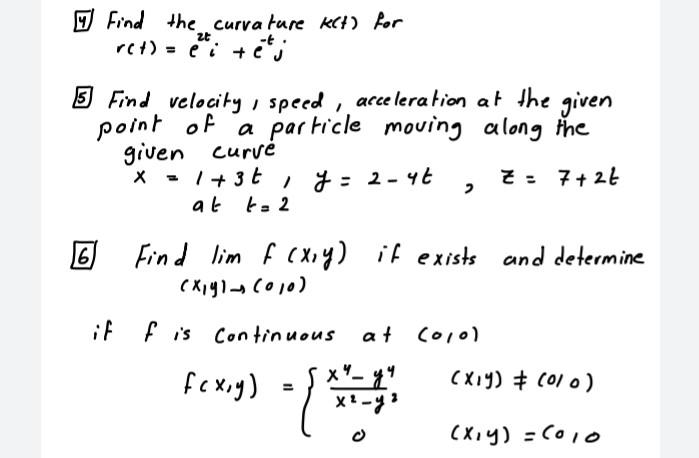### Create an Account

Already have account?

### Forgot Your Password ?

Home / Questions / Find the curvature KCH) for ret) = ei tej zt 5 Find velocity , speed, acceleration at the ...

# Find the curvature KCH) for ret) = ei tej zt 5 Find velocity , speed, acceleration at the given point of of a particle moving along the given curve X = 1 + 3t , y2-45 Z = 7+27 at to 2 16 if Find lim f

Find the curvature KCH) for ret) = ei tej zt 5 Find velocity , speed, acceleration at the given point of of a particle moving along the given curve X = 1 + 3t , y2-45 Z = 7+27 at to 2 16 if Find lim f cxoy) it exists and determine (Kg)-(a +) fis Continuous at colo) fcx,y) (Xiy) # (010) x1-12 (x,y) =6010Apr 13 2021 View more View Less

#### Answer (Solved)Subscribe To Get Solution